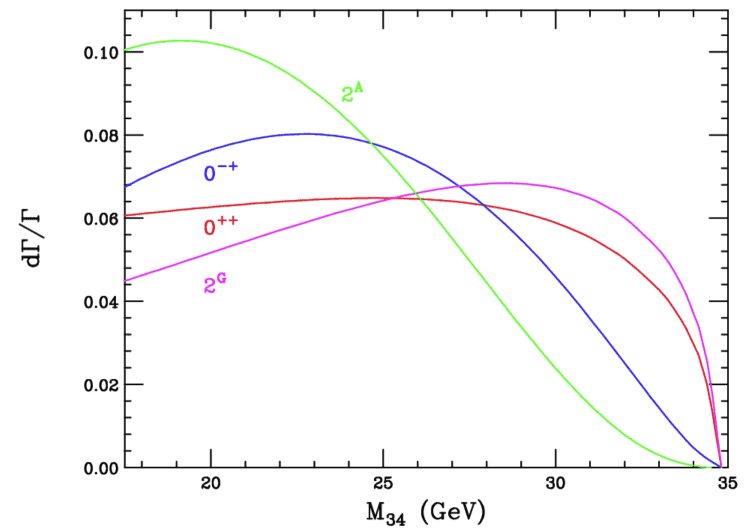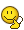# Zero spin of Higgs boson? Is it really zero?

• I
• curiuousgoerge
In summary, the Higgs boson has a zero spin due to the Higgs mechanism and the need for a non-zero vacuum expectation value. This is because other fields with spin would break symmetries. Each elementary particle has a fixed total spin, with half-integer spins for fermions and integer spins for bosons. Cooling a particle to a point where it has no intrinsic spin does not mean it would disappear, as total spin is a defining parameter for each particle.f

#### curiuousgoerge

TL;DR Summary
Zero spin of Higgs boson? Is it really zero? Where is the spin (intrinsic angular momentum) of the Higgs boson on so small that we quantify it as having zero spin?
Zero spin of Higgs boson? Is it really zero? Where is the spin (intrinsic angular momentum) of the Higgs boson on so small that we quantify it as having zero spin?

I am aware of the reduced plank constant. But I we sure there is nothing in between the reduce Planck’s constant and the zero spin of the Higgs boson?

The Higgs-boson basically has to have spin-zero in order to produce a vacuum expectation value, which was the entire point of introducing the higgs field.

•vanhees71
Where is the spin (intrinsic angular momentum) of the Higgs boson on so small that we quantify it as having zero spin?
Spin is quantized, it cannot be arbitrarily small.

•protonsarecool, ohwilleke, Lord Jestocost and 1 other person
The Higgs-boson basically has to have spin-zero in order to produce a vacuum expectation value, which was the entire point of introducing the higgs field.
Can you elaborate on that more? And was the Higgs boson zero spin theorized before it was observed confirmed or was it observed and then confirmed has zero?

Where to begin..
The original way to assign particle masses in field theory was to introduce quadratic terms in the Lagrangian, this doesn't work for gauge bosons or fermions participating in the weak interaction. To remedy this the Higgs mechanism was used to add effective mass terms to the Lagrangian via the Higgs field, in order for this to work the Higgs field has to have a non-zero vacuum expectation value. This basically means that the Higgs field cannot disappear (on average) even in its lowest energy state; there's still some of it around no matter how cold you make the system. However, because the vacuum must be lorentz invariant, so must the vacuum expectation value of any field. This means that no vector (spin-1) or spinor (spin-1/2) field can have a non-zero vacuum expectation value as it would pick out a preferred direction in space. This leaves only the scalar field (spin-0) capable of having a non-zero vacuum expectation value, as it has no direction and can do so without breaking any symmetries. Thus the higgs field was always described by a scalar field with no spin.

•ohwilleke, strangerep, Orodruin and 2 others
Where to begin..
The original way to assign particle masses in field theory was to introduce quadratic terms in the Lagrangian, this doesn't work for gauge bosons or fermions participating in the weak interaction. To remedy this the Higgs mechanism was used to add effective mass terms to the Lagrangian via the Higgs field, in order for this to work the Higgs field has to have a non-zero vacuum expectation value. This basically means that the Higgs field cannot disappear (on average) even in its lowest energy state; there's still some of it around no matter how cold you make the system. However, because the vacuum must be lorentz invariant, so must the vacuum expectation value of any field. This means that no vector (spin-1) or spinor (spin-1/2) field can have a non-zero vacuum expectation value as it would pick out a preferred direction in space. This leaves only the scalar field (spin-0) capable of having a non-zero vacuum expectation value, as it has no direction and can do so without breaking any symmetries. Thus the higgs field was always described by a scalar field with no spin.
so the higgs boson does have spin, but for formulaic reasons it is simplified as a scalar with a 0 spin?
theoretically if subatomic particle is cooled down to a point where it has no intrinsic spin, does that mean it would disappear?

so the higgs boson does have spin, but for formulaic reasons it is simplified as a scalar with a 0 spin?
theoretically if subatomic particle is cooled down to a point where it has no intrinsic spin, does that mean it would disappear?
You have misunderstood the quantisation of spin. Each elementary particle has a fixed total spin, which can be one of ##0, \frac 1 2, 1, \frac 3 2 \dots##. This value of spin is fixed, in the same way that the mass and charge of the particle is fixed - total spin is one of the defining parameters of each particle.

(In fact, particles with half-integer spins are called fermions; and, particles with integer spin are called bosons.

The Higgs Boson has zero spin. The photon has spin ##1##. The electron has spin ##\frac 1 2##.

•vanhees71 and ohwilleke
You have misunderstood the quantisation of spin. Each elementary particle has a fixed total spin, which can be one of ##0, \frac 1 2, 1, \frac 3 2 \dots##. This value of spin is fixed, in the same way that the mass and charge of the particle is fixed - total spin is one of the defining parameters of each particle.

(In fact, particles with half-integer spins are called fermions; and, particles with integer spin are called bosons.

The Higgs Boson has zero spin. The photon has spin ##1##. The electron has spin ##\frac 1 2##.
Also, worth noting, that there are many composite particles with spin-0 (e.g. a pion), and that one of the first things that was done after the discovery of the Higgs in 2012 was to confirm its zero spin nature to very high probability.

Last edited:
••Delta2 and vanhees71
Dumb question: how does one determine the spin of the Higgs boson from the experimental data generated by the LHC?

•vanhees71
theoretically if subatomic particle is cooled down to a point where it has no intrinsic spin
You cannot "cool down" elementary particles, and you cannot change their spin with any other process. You can only change the direction of their spin (if it's non-zero, otherwise there is no such direction).
Dumb question: how does one determine the spin of the Higgs boson from the experimental data generated by the LHC?
• By conservation of angular momentum. If it can decay e.g. to two bosons it has to be a boson (integer spin).
• Via the Landau-Yang theorem a massive particle decaying to two photons cannot have spin 1.
• By looking at the angular distributions of the decay particles if there are more than two. This is largely done with the decay to four muons. Different spin options lead to different distributions.

•protonsarecool, strangerep, dextercioby and 5 others
You have misunderstood the quantisation of spin. Each elementary particle has a fixed total spin, which can be one of ##0, \frac 1 2, 1, \frac 3 2 \dots##. This value of spin is fixed, in the same way that the mass and charge of the particle is fixed - total spin is one of the defining parameters of each particle.

(In fact, particles with half-integer spins are called fermions; and, particles with integer spin are called bosons.

The Higgs Boson has zero spin. The photon has spin ##1##. The electron has spin ##\frac 1 2##.
I understand the basics of 1 and 1/2 spins. and they are multiplied by the reduced Planck constant (ℏ)
Can anyone explain what biggest differences are between Pions and higg bosons when it comes to 0 spin?

I know: Pion = In particle physics, a pion is any of three subatomic particles: π⁰ , π⁺ , and π⁻ . Each pion consists of a quark and an antiquark and is therefore a meson.

How do the three subatomic particles bond to each other is there is no intrinsic spin? Or do the spins neutralize each other, creating 0 spin?

I know: Pion = In particle physics, a pion is any of three subatomic particles: π⁰ , π⁺ , and π⁻ . Each pion consists of a quark and an antiquark and is therefore a meson.

How do the three subatomic particles bond to each other is there is no intrinsic spin? Or do the spins neutralize each other, creating 0 spin?
If an up quark and a down anti-quark combine (both are spin 1/2, as you know), their total spin must be 0 or 1. Depending on whether the spins align or not. If the spins align, you get a spin 1 particle (##\rho##) and if the spins cancel you get a spin 0 particle (pion).

Note that total spin is one of the defining characteristics of each particle. It's not a variable dynamic quantity like it is in classical physics.

•vanhees71, mfb and ohwilleke
Spin is quantized, it cannot be arbitrarily small.

What is the minimal spin of a Lorentz-scalar field then?

There is `no question that the Higgs boson has spin 0 (and even parity). The experiments do all sorts of fancy-schmancy fitting, but the reason is quite simple: the 125 GeV of the Higgs mass has to go somewhere. In the ZZ* decay, the on-shell Z takes 90 GeV, and the rest is split between the off-shell Z mass and kinetic energy, which is L2/2I. So the off-shell Z mass distribution changes with the Higgs spin. See Boughezal et al.The fitters, of course. rediscover this relationship when they are run.

There is no room for doubt about the quantum numbers of the Higgs.

Last edited:
•ohwilleke and mfb
What is the minimal spin of a Lorentz-scalar field then?
Exactly zero, which is not arbitrarily small.

••protonsarecool, ohwilleke and malawi_glenn
Exactly zero, which is not arbitrarily small.
I read totally wrong there, my bad•ohwilleke and Demystifier
What is the minimal spin of a Lorentz-scalar field then?
By definition the spin of a scalar field is zero. The spin quantum number ##s## defines the representation of rotations for the states of 0 momentum (massive particles; for massless ones it's a bit more complicated).

If you mean total angular momentum, which is the only angular momentum that's observable in relativistic physics, the minimal ##J## is zero too for scalar fields.

•ohwilleke